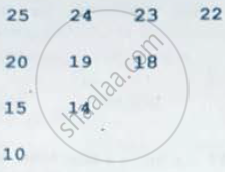Share

# Write a Definition for a Function Upperhalf(Int Mat) in C++ Which Displays the Elements in the Same Way as per the Example Is Shown Below. Thew Function Should Display The Content in the Following Format: - CBSE (Science) Class 12 - Computer Science (C++)

ConceptOne and Two Dimensional Arrays - Sequential Allocation and Address Calculation

#### Question

Write a definition for a function UpperHalf(int Mat) in C++ which displays the elements in the same way as per the example is shown below.

For example, if the content of the array Mat is as follows:

 25 24 23 22 20 19 18 17 15 14 13 12 10 9 8 7

Thew function should display the content in the following format:#### Solution

void UpperHalf(int Mat)
{
for (int i = 0; i < 4; i++)
{
for (int j = 0; j < 4; j++)
{
cout<< Mat[i][j] <<"";
}
cout<< endl;
}
}
Is there an error in this question or solution?

#### APPEARS IN

Solution Write a Definition for a Function Upperhalf(Int Mat) in C++ Which Displays the Elements in the Same Way as per the Example Is Shown Below. Thew Function Should Display The Content in the Following Format: Concept: One and Two Dimensional Arrays - Sequential Allocation and Address Calculation.
S